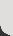﻿ Exploring division and multiplication - (TLF R11083 v3.0.0)

This collection of 17 digital curriculum resources contains activities to consolidate and extend students' problem-solving skills in the areas of multiplication and division. The first category, arrays, allows students to visualise problems while consolidating the idea that multiplication involves repeated groups of objects. The second category, solving multiplication and division problems, enables students to solve and create problems and helps them to develop their own strategies for solving equations. Assessment tools are included.

L106 The array

L2056 Pobble arrays: make multiples

L2058 Pobble arrays: find two factors

L2059 Arrays: factor families

L2060 Arrays: explore factors

L83 The multiplier: generate easy multiplications

L2007 The divider: without remainders

L2009 The divider: solve your own problem

L2008 The divider: whole number remainders